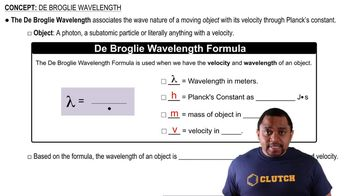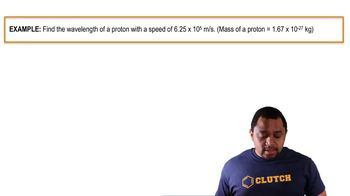Start typing, then use the up and down arrows to select an option from the list.
6:11 minutes
Problem 133
Textbook Question

# X rays with a wavelength of 1.54 * 10-10 m are produced when a copper metal target is bombarded with high-energy electrons that have been accelerated by a voltage difference of 30,000 V. The kinetic energy of the electrons equals the product of the voltage difference and the electronic charge in coulombs, where 1 volt-coulomb = 1 J. (a) What is the kinetic energy in joules and the de Broglie wavelength in meters of an electron that has been accel-erated by a voltage difference of 30,000 V?Verified Solution
This video solution was recommended by our tutors as helpful for the problem above.
86views